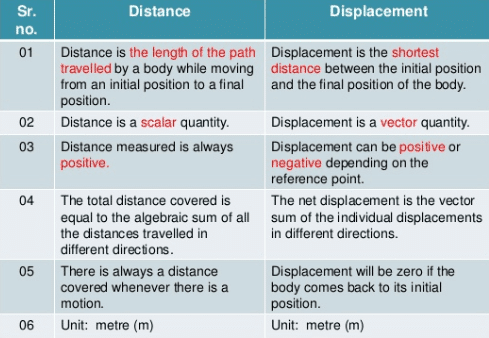Mechanics

# Differentiate Between Distance And DisplacementThe basic difference between Distance and displacement is that distance is the length of a path between two points and displacement is the shortest distance between two points.
More differences between distance Vs displacement are tabulated below.
You may also be interested in the: Difference between speed and velocity

## What is Displacement?

Displacement is considered a vector magnitude and we can describe it as the variation of the position of a body. The displacement focuses on the length of the path of an object that exists considering an initial point and another endpoint. It can be said that the result is a straight line between these two points.
Therefore, it should be noted that the displacement does not depend on the path followed by the body in question but as mentioned above the difference between the points considered as initial and final.
For example:

1. If the same person in example # 1, take the tour described above where he leaves his house, travels through a series of places during the day, and finally returns home, considering an initial point like his house and an endpoint his house again, the total displacement of the day will have been 0 meters because the starting and ending point is the same.

However, if the displacement of the person from his house to the market is considered, the displacement of the person for that place would be 300 meters.

## What is Distance?

Distance is the length of a path between two points. It is denoted by “S”. It is a scalar quantity.
Also read: Motion & its types

### Distance and Displacement Difference

For all the above, we are able to distinguish distance and displacement in tabular form as:

 Distance Displacement It is a magnitude that measures the length that is traveled by an object from one point to another. It is a magnitude that measures the variation of the position of a body between two points, considering a starting point and an endpoint. It is considered a scalar magnitude. It is considered a vector magnitude. It depends on the path that the object follows. It does not depend on the path that the object follows. It is expressed by a number and a unit of magnitude frequently in meters. In physics, it is expressed by a module (value), unity, direction, and meaning. It is obtained from the sum of all the lengths traveled by an object. It is obtained from the difference in the length value of an endpoint and an initial point of an object.

Related topics

External source
https://byjus.com/physics/difference-between-distance-and-displacement/